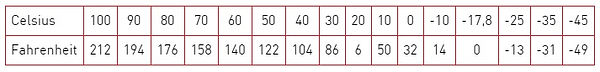top of page

## TABELAS DE CONVERSÃO

Escala de Temperaturas

To compute Fahrenheit: Multiply Centigrade by 1.8 and add 32 To compute Centigrade: Substract 32 from Fahrenheit and divide by 1.8

Pesos e Medidas

Medidas de pesos
1 grain = 0.06 grams
1 dram = 1.77 grams
1 ounce (16 drachms)= 28.35 grams
1 pound (16 ounces) = 0.45 kilogram
1 stone = 6.35 kilogram
1 quarter ( = 28 lbs.) = 12,70 kilos
1 hundredweight (cwt.) = 112 lbs. = 50.80 kilogram
1 ton (20 cwt.) = 1016 kilos

1 cubic inch = 16.39 cm³
1 cubic foot (1728 cub.in.) = 0.03 m³
1 cubic yard (27 cub.feet) = 0.76 m³
1 gill = 1.42 decilitre
1 pint (4 gills) = 0.57 litre
1 quart (2 pints) = 1.4 litre
1 gallon (4 quarts) = 4.55 litre
1 peck (2 gallons) = 9.09 litre
1 bushel (8 gallons) = 3.64 decalitre
1 quarter (8 bushells) = 2.90 hectolitre
1 cm³ = 0.06 cubic inch
1 dm³ = 61.02 cubic inch
1 m³ = 35.32 cubic feet or 1.31 cubic yards
1 centilitre = 0.07 gill
1 decilitre = 0.17 pint
1 litre = 1.76 pint
1 decalitre = 2.2 gallons
1 hectolitre = 2.75 bushels

Medidas de comprimento
1 inch = 25.40 mm
1 foot (12 inches) = 0.30 m
1 yard (3 feet) = 0.91 m
1 fathom (6 feet) = 1.83 m
1 pole (5½ yards) = 5.03 m
1 chaim (22 yards) = 20.12 m
1 furlong (220 yard
s) = 201.17 m
1 mile (8 furlongs) = 1609 m
1 geographical (sea or nautical) mile = 1855 m

Medidas de superfície
1 square inch = 6.45 cm²
1 square foot (144 sq. inches) = 9.20 dm²
1 square yard (9 sq. feet) = 0.84 m²
1 perch (30 ½ sq. yards) = 25.29 m²
1 rood (40 perches) = 10.11 aren
1 acre (4840 sq. yards) = 4047 m²
1 square mile (640 acres) = 259 hectares
1 cm² = 0.15 square inch
1 dm² = 15.50 square inch
1 m² = 10.76 sq. feet or 1.20 sq. yards
1 are = 119.60 square yards
1 hectare = 2.47 acresbottom of page